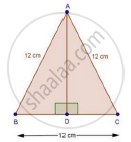Share

Books Shortlist
Your shortlist is empty

# Solution for Calculate the Height of an Equilateral Triangle Each of Whose Sides Measures 12 Cm. - CBSE Class 10 - Mathematics

ConceptApplication of Pythagoras Theorem in Acute Angle and Obtuse Angle

#### Question

Calculate the height of an equilateral triangle each of whose sides measures 12 cm.

#### SolutionWe have,

ΔABC is an equilateral Δ with side 12 cm.

Draw AE ⊥ BC

In ΔABD and ΔACD

AB = AC [Each 12 cm]

Then, ΔABD ≅ ΔACD [By RHS condition]

∴ AD2 + BD2 = AB2

⇒ AD2 + 62 = 122

⇒ AD2 = 144 − 36 = 108

⇒ AD = sqrt108 = 10.39 cm

Is there an error in this question or solution?

#### Video TutorialsVIEW ALL 

Solution for question: Calculate the Height of an Equilateral Triangle Each of Whose Sides Measures 12 Cm. concept: Application of Pythagoras Theorem in Acute Angle and Obtuse Angle. For the course CBSE
S